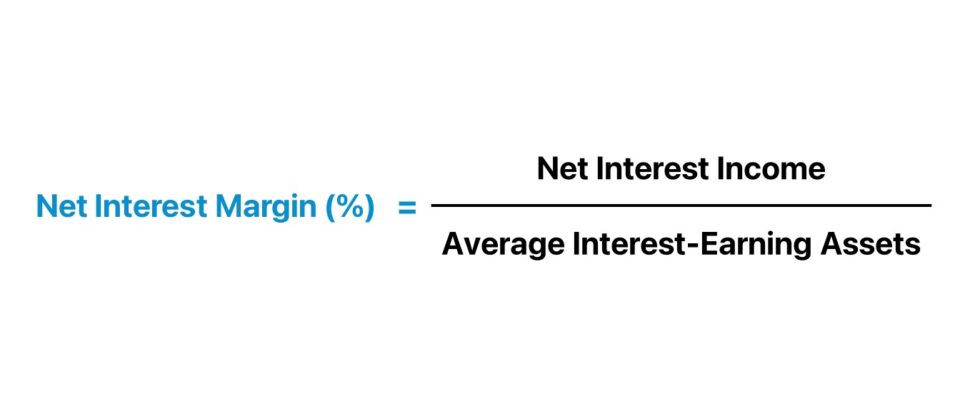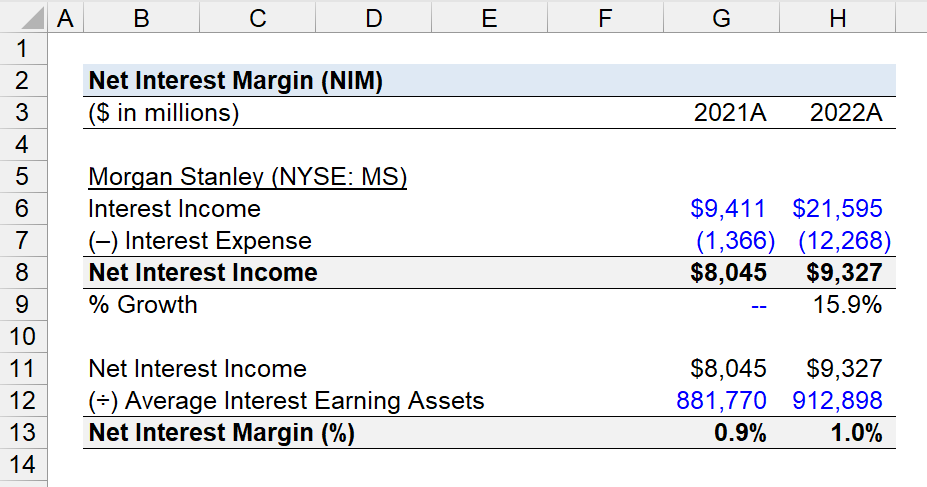Welcome to Wall Street Prep! Use code at checkout for 15% off.# Net Interest Margin (NIM)

Guide to Understanding Net Interest Margin (NIM)## How to Calculate Net Interest Margin?

The net interest margin (NIM) is a measure of profitability in the financial sector, which comprises financial institutions such as commercial and corporate banks and other lenders.

The lending business model of a bank or financial institution is oriented around structuring debt products, such as loans and bonds, to consumer or corporate borrowers in exchange for periodic interest payments across the borrowing term and the repayment of the original debt principal at maturity.

There are two parts to the structure of the traditional lending business model:

1. Interest Income → The lender earns interest on their loan portfolio, in which debt is provided to borrowers in exchange for interest payments (“inflow”).
2. Interest Expense → The lender must pay Interest on customer deposits, which aside from protection of funds, is the incentive for customers to open and maintain an active checking or savings account (“outflow”).

In terms of the lending portfolio of a bank, interest-earning assets include primarily bank loans, mortgages, and other financing products.

In contrast, the interest-bearing liabilities within the portfolio consist of customer deposits and borrowings from other banks.

The difference between the interest income and interest expense of a bank or financial institution results in the net interest income (NII) metric.

Net Interest Income (NII) = Interest Income  Interest Expense

The net interest income (NII) is a comparison between the income generated from interest-earning assets to the interest expense burden incurred from interest-bearing liabilities.

Conceptually, the net interest margin (NIM) reflects the percent net interest “spread” on the loan portfolio.

The net interest income (NII) must be a positive value – where interest income exceeds interest expense – for the lending model to be sustainable over the long term.

The step-by-step process to calculate the net interest margin (NIM) is as follows.

1. Calculate Net Interest Income (NII)
2. Determine Average Interest-Bearing Assets in Loan Portfolio
3. Divide Net Interest Income (NII) by the Average Interest-Bearing Assets in the Loan Portfolio
4. Multiply the Resulting Figure by 100 to Convert into a Percentage

## Net Interest Margin Formula

The formula to calculate the net interest margin (NIM) is as follows.

Net Interest Margin (NIM) = Net Interest Income (NII) ÷ Average Interest-Earning Assets

Where:

• Net Interest Income (NII) = Interest Income – Interest Expense
• Average Interest-Earning Assets = (Beginning + Ending Interest-Earning Assets) ÷ 2

## Net Interest Margin Calculator

We’ll now move to a modeling exercise, which you can access by filling out the form below.#### Get the Excel Template!Submitting...

## Net Interest Margin Calculation Example (NIM)

Suppose we’re tasked with calculating the net interest margin (NIM) of Morgan Stanley (NYSE: MS) for fiscal years ending 2021 and 2022.

In 2022, the average

Net Interest Margin (NIM)
(\$ in millions) 2021A 2022A
Morgan Stanley (NYSE: MS)
Interest Income \$9,411 \$21,595
(–) Interest Expense (1,366) (12,268)
Net Interest Income \$8,045 \$9,327
% Growth 15.9%

The financial data was sourced from the following section of Morgan Stanley’s 10-K.

The net interest income (NII) of Morgan Stanley was \$8,045 million and \$9,327 million in 2021 and 2022, respectively.

• Net Interest Income (NII) – 2021A = \$9,411 million – \$1,366 million = \$8,045 million
• Net Interest Income (NII) – 2022A = \$21,595 million – \$12,268 million = \$9,327 million

The year-over-year (YoY) growth rate in the net interest income (NII) of Morgan Stanley was 15.9%, which was attributable to the rise in the market interest rate.

• NII % Growth Rate = (\$9,327 million ÷ \$8,045 million) – 1 = 15.9%

By dividing the net interest income (NII) by the average interest-bearing assets in the corresponding period, we can determine the net interest margin (NIM).

• Net Interest Margin (NIM) – 2021A = \$8,045 million ÷ \$881,770 million = 0.9%
• Net Interest Margin (NIM) – 2022A = \$9,327 million ÷ \$912,898 million = 1.0%Step-by-Step Online Course

### Everything You Need To Master Financial Modeling

Enroll in The Premium Package: Learn Financial Statement Modeling, DCF, M&A, LBO and Comps. The same training program used at top investment banks.